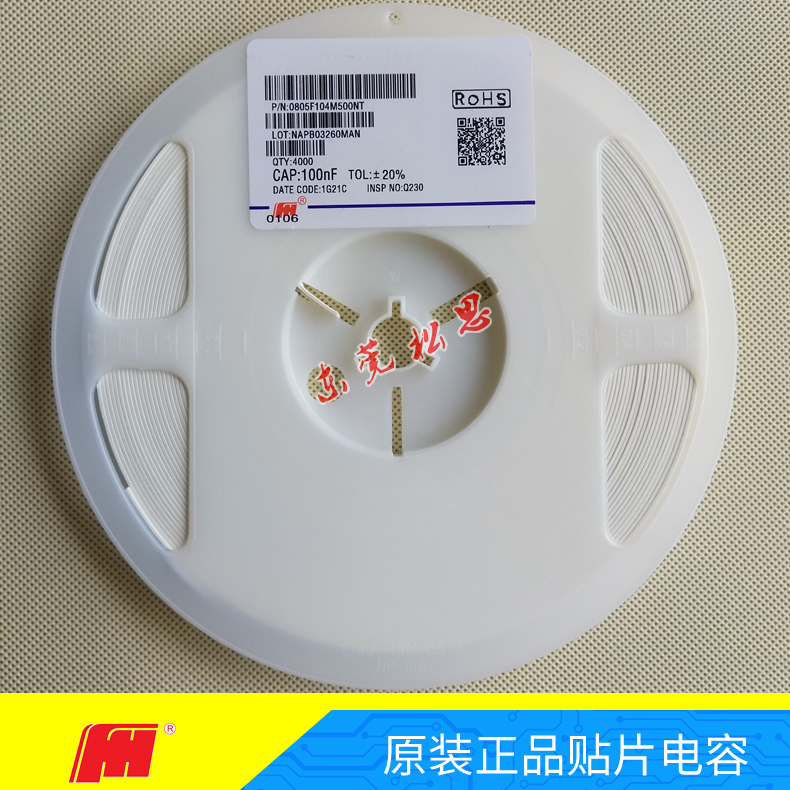0769-87868126

E-mail：allsmd@126.com

# 电容的单位怎么换算

1法拉（F）= 1000毫法（mF）=1000000微法（μF）；

1微法（μF）= 1000纳法（nF）= 1000000皮法（pF）。

105=1000000pF=1000nF= 1 μF

104=100000pF  =100nF  =0.1 μF

103=10000pF    =10nF    = 0.01 μF

102=1000pF      =1nF      =0.001 μF

224=22 * 10^4pF=220000pF=220nF=0.22uF